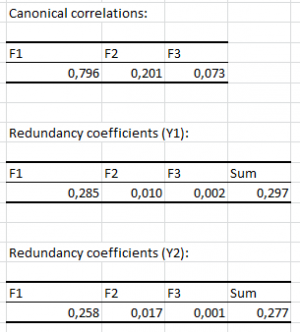# Canonical Correlation Analysis (CCorA)

Canonical Correlation Analysis is used to test the correlation between two sets of variables. Available in Excel using the XLSTAT add-on statistical software.## Origins and aim of Canonical Correlation Analysis

Canonical Correlation Analysis (CCorA, sometimes CCA, but we prefer to use CCA for Canonical Correspondence Analysis) is one of the many statistical methods that allow studying the relationship between two sets of variables.It studies the correlation between two sets of variables and extract from these tables a set of canonical variables that are as much as possible correlated with both tables and orthogonal to each other.

Discovered by Hotelling (1936) this method is used a lot in ecology but is has been supplanted by RDA (Redundancy Analysis) and by CCA (Canonical Correspondence Analysis).

## Principles of Canonical Correlation Analysis

This method is symmetrical, contrary to RDA, and is not oriented towards prediction. Let Y1 and Y2 be two tables, with respectively p and q variables. Canonical Correlation Analysis aims at obtaining two vectors a(i) and b(i) such that

ρ(i) = cor[Y1a(i),Y2b(i)] = cov(Y1a(i) Y2b(i)) / [var(Y1a(i)).var(Y2b(i))]

is maximized. Constraints must be introduced so that the solution for a(i) and b(i) is unique. As we are in the end trying to maximize the covariance between Y1a(i) and Y2b(i) and to minimize their respective variance, we might obtain components that are well correlated among each other, but that are not explaining well Y1 and Y2. Once the solution has been obtained for i=1, we look for the solution for i=2 where a(2) and b(2) must respectively be orthogonal to a(1) and b(2), and so on. The number of vectors that can be extracted is to the maximum equal to min(p, q).

Note: The inter-batteries analysis of Tucker (1958) is an alternative where one wants to maximize the covariance between the Y1a(i) and Y2b(i) components.

## Results for Canonical Correlation Analysis in XLSTAT

• Similarity matrix: . The matrix that corresponds to the "type of analysis" chosen in the dialog box is displayed.
• Eigenvalues and percentages of inertia: In this table are displayed the eigenvalues, the corresponding inertia, and the corresponding percentages. Note: in some software, the eigenvalues that are displayed are equal to L / (1-L), where L is the eigenvalues given by XLSTAT.
• Wilks Lambda test: This test allows to determine if the two tables Y1 and Y2 are significantly related to each canonical variable.
• Canonical correlations: The canonical correlations, bounded by 0 and 1, are higher when the correlation between Y1 and Y2 is high. However, they do not tell to what extent the canonical variables are related to Y1 and Y2. The squared canonical correlations are equal to the eigenvalues and, as a matter of fact, correspond to the percentage of variability carried by the canonical variable.

The results listed below are computed separately for each of the two groups of input variables.

• Redundancy coefficients: These coefficients allow to measure for each set of input variables what proportion of the variability of the input variables is predicted by the canonical variables.
• Canonical coefficients: These coefficients (also called Canonical weights, or Canonical function coefficients) indicate how the canonical variables were constructed, as they correspond to the coefficients in the linear combine that generates the canonical variables from the input variables. They are standardized if the input variables have been standardized. In that case, the relative weights of the input variables can be compared.
• Correlations between input variables and canonical variables: Correlations between input variables and canonical variables (also called Structure correlation coefficients, or Canonical factor loadings) allow understanding how the canonical variables are related to the input variables.
• Canonical variable adequacy coefficients: The canonical variable adequacy coefficients correspond, for a given canonical variable, to the sum of the squared correlations between the input variables and canonical variables, divided by the number of input variables. They give the percentage of variability taken into account by the canonical variable of interest.
• Square cosines: The squared cosines of the input variables in the space of canonical variables allow to know if an input variable is well represented in the space of the canonical variables. The squared cosines for a given input variable sum to 1. The sum over a reduced number of canonical axes gives the communality.
• Scores: The scores correspond to the coordinates of the observations in the space of the canonical variables.### analyze your data with xlstat

14-day free trial

Included in

Related features